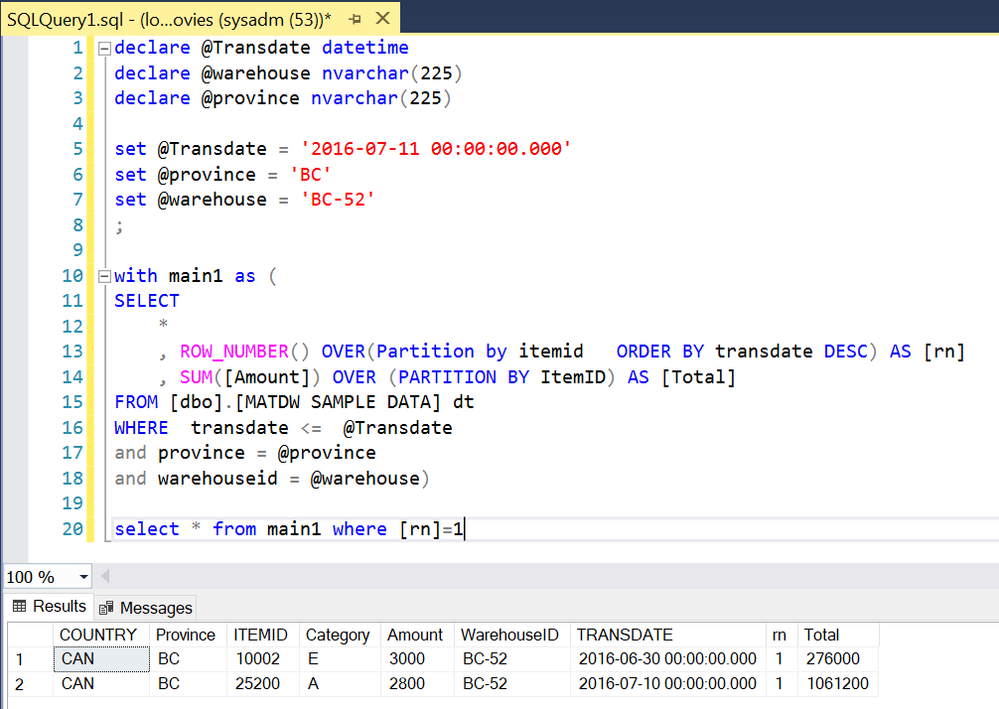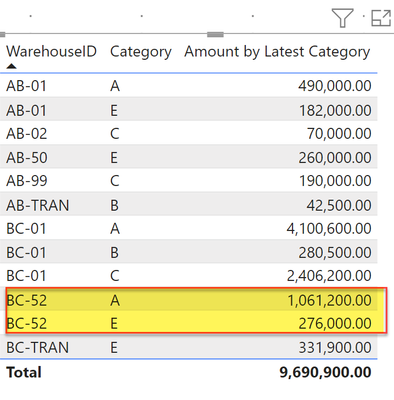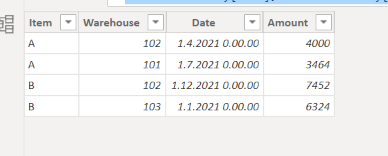cancel
Showing results for
Did you mean:Helper V

## SQL to DAX conversion

I am trying to get the equivalent logic in DAX for below SQL

declare @date datetime
declare @warehouse nvarchar(225)
declare @province nvarchar(225)

set @Transdate = '2021-09-18 00:00:00.000'
set @province = 'BC'
set @warehouse = 'BC-01'

----- THIS CTE RANKS THE ITEMS TO GET THE MOST RECENT category -----------
with main1 as (
SELECT * ,
ROW_NUMBER() OVER(Partition by itemid, warehouseid ORDER BY transdate DESC) AS rn
FROM [EDW].[FACT].[InventoryBalancedet_2] dt
WHERE province = @province
and warehouseid = @warehouse
and transdate <= @Transdate
),

------ THIS CTE RANKS amount of an item by warehouse -----------

main2 as (
select sum(Amount) as AMOUNT
, ItemID
,warehouseid

FROM [EDW].[FACT].[InventoryBalancedet_2]
WHERE province = @province
and warehouseid = @warehouse
and transdate <= @Transdate
group by ItemID , warehouseid
),

------------ COMBINING THE QUERIES TOGETHER --------------------
main3 as (
SELECT m2.AMOUNT, m2.warehouseid , m1.Category
FROM
main1 m1
inner join main2 m2 on m1.warehouseid = m2.warehouseid
and m1.ItemID = m2.ItemID
and m1.rn = 1
)

select sum(m3.total) ,m3.Category
FROM main3 m3
group by m3.Category

1 ACCEPTED SOLUTIONSuper User

Happy New Year, pal! Thanks for tagging me for some tricky game of DAX.

I'm afraid you were mislead by PO's sql; first of all, I simplied it this way,

``````declare @Transdate datetime
declare @warehouse nvarchar(225)
declare @province nvarchar(225)

set @Transdate = '2016-07-11 00:00:00.000'
set @province = 'BC'
set @warehouse = 'BC-52'
;

with main1 as (
SELECT
*
, ROW_NUMBER() OVER(Partition by itemid   ORDER BY transdate DESC) AS [rn]
, SUM([Amount]) OVER (PARTITION BY ItemID) AS [Total]
FROM [dbo].[MATDW SAMPLE DATA] dt
WHERE  transdate <=  @Transdate
and province = @province
and warehouseid = @warehouse)

select * from main1 where [rn]=1``````DAX

``````Total =
VAR __cat = MAX( 'MATDW SAMPLE DATA'[Category] )
VAR __summary =
CALCULATETABLE(
SUMMARIZE(
'MATDW SAMPLE DATA',
'MATDW SAMPLE DATA'[ITEMID],
'MATDW SAMPLE DATA'[WarehouseID]
),
"@amt", CALCULATE( SUM( 'MATDW SAMPLE DATA'[Amount] ) ),
"@maxdate", CALCULATE( MAX( 'MATDW SAMPLE DATA'[TRANSDATE] ) )
),
"@Cat",
VAR __dt = [@maxdate]
RETURN
CALCULATE(
MAX( 'MATDW SAMPLE DATA'[Category] ),
'MATDW SAMPLE DATA'[TRANSDATE] = __dt
)
),
ALLSELECTED( 'MATDW SAMPLE DATA'[Category] )
)
RETURN
IF(
__cat IN SELECTCOLUMNS( __summary, "_cat", [@Cat] ),
SUMX( FILTER( __summary, [@Cat] = __cat ), [@amt] )
)``````Thanks to the great efforts by MS engineers to simplify syntax of DAX! Most beginners are SUCCESSFULLY MISLED to think that they could easily master DAX; but it turns out that the intricacy of the most frequently used RANKX() is still way beyond their comprehension! DAX is simple, but NOT EASY!
10 REPLIES 10Super User

@CNENFRNL well done, but one thing is missing if you turn on the total that doesn't look correct and tht's why in my measure I have to create a final measure to take care of the total. FYI

Subscribe to the @PowerBIHowTo YT channel for an upcoming video on List and Record functions in Power Query!!

Learn Power BI and Fabric - subscribe to our YT channel - Click here: @PowerBIHowTo

If my solution proved useful, I'd be delighted to receive Kudos. When you put effort into asking a question, it's equally thoughtful to acknowledge and give Kudos to the individual who helped you solve the problem. It's a small gesture that shows appreciation and encouragement! ❤

Did I answer your question? Mark my post as a solution. Proud to be a Super User! Appreciate your Kudos 🙂
Feel free to email me with any of your BI needs.Super User

@smpa01 wow it is pretty challenging, I did make it thru but have to revisit some point in time. Here are the measures which will take care of it :

``````Category for Item =
MAXX (
SUMMARIZE (
'MATDW SAMPLE DATA',
'MATDW SAMPLE DATA'[WarehouseID],
'MATDW SAMPLE DATA'[ITEMID],
'MATDW SAMPLE DATA'[TRANSDATE],
"@MaxDt", CALCULATE (
MAX ( 'MATDW SAMPLE DATA'[TRANSDATE] ),
ALLSELECTED ('MATDW SAMPLE DATA'[TRANSDATE] )
)
),
IF (
'MATDW SAMPLE DATA'[TRANSDATE] = [@MaxDt],
CALCULATE ( MAX ('MATDW SAMPLE DATA'[Category] ) )
)
)

Amount by Latest Category (Internal) =
VAR __table =
SUMMARIZE (
'MATDW SAMPLE DATA',
'MATDW SAMPLE DATA'[WarehouseID],
'MATDW SAMPLE DATA'[ITEMID]
// ,"@Sum", SUM ('MATDW SAMPLE DATA'[Amount] )
),
"@LatestCategory", CALCULATE ( [Category for Item], REMOVEFILTERS ( 'MATDW SAMPLE DATA'[Category] ) ),
"@CurrentVisibleCategory", CALCULATE (  MAX ('MATDW SAMPLE DATA'[Category] ) )
)
// VAR __currentVisibleCategory = MAX ( 'MATDW SAMPLE DATA'[Category] )
RETURN
SUMX (
__table,
VAR __latestCategory = [@LatestCategory]
VAR __currentVisibleCategory = [@CurrentVisibleCategory]
RETURN
IF ( __latestCategory = __currentVisibleCategory,
CALCULATE (
SUM ( 'MATDW SAMPLE DATA'[Amount] ),
ALLSELECTED ( 'MATDW SAMPLE DATA'[Category] )
)
)
)

Amount by Latest Category =
IF (
HASONEFILTER ('MATDW SAMPLE DATA'[Category] ),
[Amount by Latest Category (Internal)],
SUMX (
SUMMARIZE (
'MATDW SAMPLE DATA',
'MATDW SAMPLE DATA'[WarehouseID],
'MATDW SAMPLE DATA'[Category]
),
"@Amt", [Amount by Latest Category (Internal)]
),
[@Amt]
)
)``````

and here is the output:Learn about conditional formatting at Microsoft Reactor

My latest blog post The Power of Using Calculation Groups with Inactive Relationships (Part 1) (perytus.com) I would  Kudos if my solution helped. 👉 If you can spend time posting the question, you can also make efforts to give Kudos to whoever helped to solve your problem. It is a token of appreciation!

Visit us at https://perytus.com, your one-stop-shop for Power BI-related projects/training/consultancy.

Subscribe to the @PowerBIHowTo YT channel for an upcoming video on List and Record functions in Power Query!!

Learn Power BI and Fabric - subscribe to our YT channel - Click here: @PowerBIHowTo

If my solution proved useful, I'd be delighted to receive Kudos. When you put effort into asking a question, it's equally thoughtful to acknowledge and give Kudos to the individual who helped you solve the problem. It's a small gesture that shows appreciation and encouragement! ❤

Did I answer your question? Mark my post as a solution. Proud to be a Super User! Appreciate your Kudos 🙂
Feel free to email me with any of your BI needs.Super User

@parry2k  Happy new year !!! and many thanks for taking time out to look into this. Wil review later and let you know.

Proud to be a Super User!

New Animated Dashboard: Sales CalendarSuper User

@amikm can you provide a SQL DDL? or at least provide a sizeable sample of [InventoryBalancedet_2] in a pbix, upload that in a g drive/1 drive and share the link here?

Proud to be a Super User!

New Animated Dashboard: Sales CalendarHelper V

Thanks  @smpa01 , Please find the sample data and file

i have attached a zip file containing the zip file & the sql query .

i will like to get the results (Amount by category) as shown in the query .

Also i will like to use the filters below on the result set
1) transdate
2) warehouse
3) provinceSuper User

@amikm  I deleted my earlier answer cause I realized it was wrong.

I spent some time with this but could not make it to work, looping @parry2k @AlexisOlson @CNENFRNL

@parry2k @AlexisOlson @CNENFRNL  OP has a TSQL query like following

``````declare @Transdate datetime
declare @warehouse nvarchar(225)
declare @province nvarchar(225)

set @Transdate = '2016-07-11 00:00:00.000'
set @province = 'BC'
set @warehouse = 'BC-52'

----- THIS CTE  RANKS THE ITEMS TO GET THE MOST RECENT category -----------
;with main1 as (
SELECT  * ,
ROW_NUMBER() OVER(Partition by itemid, warehouseid   ORDER BY transdate DESC) AS rn
FROM [newtest].[dbo].[MATDW SAMPLE DATA] dt
WHERE  transdate <=  @Transdate
and province = @province
and warehouseid = @warehouse

)
,main2 as (
select sum(Amount) as AMOUNT
, ItemID
,warehouseid

FROM [newtest].[dbo].[MATDW SAMPLE DATA]
WHERE  transdate <=  @Transdate
and province = @province
and warehouseid = @warehouse

group by ItemID , warehouseid
)

,main3 as (
SELECT m2.AMOUNT, m2.warehouseid , m1.Category
FROM
main1 m1
inner join main2 m2 on m1.warehouseid = m2.warehouseid
and m1.ItemID = m2.ItemID
and m1.rn = 1
)
,main4 as (select sum(m3.AMOUNT)  AS AMOUNT ,m3.Category
FROM main3 m3
group by  m3.Category )

select * from main3 /*where rn=1*/

--select *
--FROM [newtest].[dbo].[MATDW SAMPLE DATA]
--WHERE  transdate <=  @Transdate
--and province = @province
--and warehouseid = @warehouse and ITEMID=10000``````

which produces thisThe equivalent DAX query for a dervied table would be following

``````Table =
VAR _basicFilterDate =
DATE ( 2016, 7, 11 )
VAR _basicFilterProvince = "BC"
VAR _basicFilterWarehouse = "BC-52"
VAR _filteredTable =
FILTER (
'MATDW SAMPLE DATA',
'MATDW SAMPLE DATA'[Province] = _basicFilterProvince
&& 'MATDW SAMPLE DATA'[TRANSDATE] <= _basicFilterDate
&& 'MATDW SAMPLE DATA'[WarehouseID] = _basicFilterWarehouse
)
var _filter = FILTER(_date,[TRANSDATE]=[maxDT])
var _groupBY = SUMMARIZE(_filter,[Category],[sum])
var _aggregation = GROUPBY(_groupBY,[Category],"_sum",SUMX(CURRENTGROUP(),[sum]))
RETURN _aggregation``````

which returns thisHowever, how can this be converted to measure that reponses to a slicer

``````VAR _basicFilterDate =
DATE ( 2016, 7, 11 )
VAR _basicFilterProvince = "BC"
VAR _basicFilterWarehouse = "BC-52"``````

Created a pbix for your convenience as it has bothered me for a while.

Proud to be a Super User!

New Animated Dashboard: Sales CalendarSuper User

Happy New Year, pal! Thanks for tagging me for some tricky game of DAX.

I'm afraid you were mislead by PO's sql; first of all, I simplied it this way,

``````declare @Transdate datetime
declare @warehouse nvarchar(225)
declare @province nvarchar(225)

set @Transdate = '2016-07-11 00:00:00.000'
set @province = 'BC'
set @warehouse = 'BC-52'
;

with main1 as (
SELECT
*
, ROW_NUMBER() OVER(Partition by itemid   ORDER BY transdate DESC) AS [rn]
, SUM([Amount]) OVER (PARTITION BY ItemID) AS [Total]
FROM [dbo].[MATDW SAMPLE DATA] dt
WHERE  transdate <=  @Transdate
and province = @province
and warehouseid = @warehouse)

select * from main1 where [rn]=1``````DAX

``````Total =
VAR __cat = MAX( 'MATDW SAMPLE DATA'[Category] )
VAR __summary =
CALCULATETABLE(
SUMMARIZE(
'MATDW SAMPLE DATA',
'MATDW SAMPLE DATA'[ITEMID],
'MATDW SAMPLE DATA'[WarehouseID]
),
"@amt", CALCULATE( SUM( 'MATDW SAMPLE DATA'[Amount] ) ),
"@maxdate", CALCULATE( MAX( 'MATDW SAMPLE DATA'[TRANSDATE] ) )
),
"@Cat",
VAR __dt = [@maxdate]
RETURN
CALCULATE(
MAX( 'MATDW SAMPLE DATA'[Category] ),
'MATDW SAMPLE DATA'[TRANSDATE] = __dt
)
),
ALLSELECTED( 'MATDW SAMPLE DATA'[Category] )
)
RETURN
IF(
__cat IN SELECTCOLUMNS( __summary, "_cat", [@Cat] ),
SUMX( FILTER( __summary, [@Cat] = __cat ), [@amt] )
)``````Thanks to the great efforts by MS engineers to simplify syntax of DAX! Most beginners are SUCCESSFULLY MISLED to think that they could easily master DAX; but it turns out that the intricacy of the most frequently used RANKX() is still way beyond their comprehension! DAX is simple, but NOT EASY!Super User

@CNENFRNL happy new year !!! Thanks for taking time out to look into this. Will review and rep on or after 2nd Jan, 22.

Proud to be a Super User!

New Animated Dashboard: Sales CalendarSuper User

Hi,

So if I understood corectly you want to get the latest warehouse values in a calculated table which is in essence a summarized version of your InventoryBalancedet_2 table?

Test data:Dax (Table):

LatestHistory =
SUMMARIZE(filter(WarehouseHistory,WarehouseHistory[Date]=calculate(MAX(WarehouseHistory[Date]),ALLEXCEPT(WarehouseHistory,WarehouseHistory[Item],WarehouseHistory[Warehouse]))),

WarehouseHistory[Item],WarehouseHistory[Warehouse],WarehouseHistory[Date],"Amount",SUM(WarehouseHistory[QTY]))

End result:I hope this helps and if it does consider accepting this as a solution and giving the post a thumbs up!

Proud to be a Super User!Super User

Is it possible for you to save this as a stored procedure on the SQL Server (or view, but you'll need to do away with the declared variables),

Then call it in Power Query?

Not only would it save you the trouble of re-creating your hard work, it would also move that transformation to to source, which is preferable.

-------------------------
Data analyst by day, hockey goalie by night.
Did I help? Then please hit that "kudos" or "accept as a solution" button!